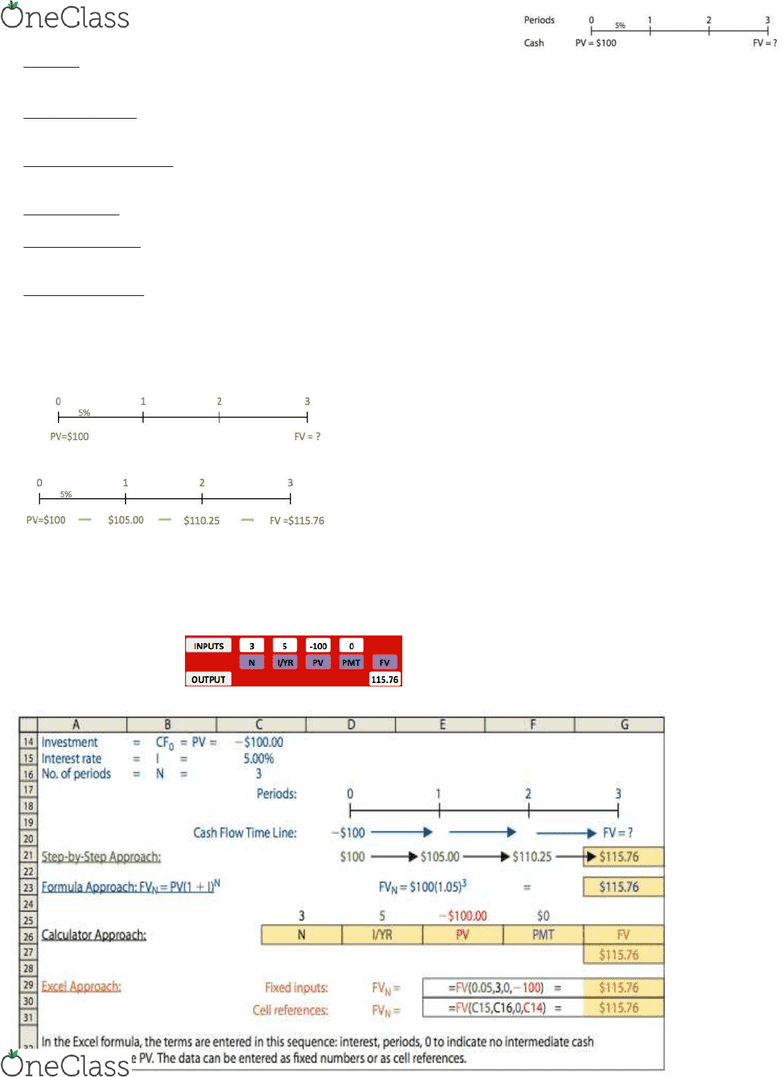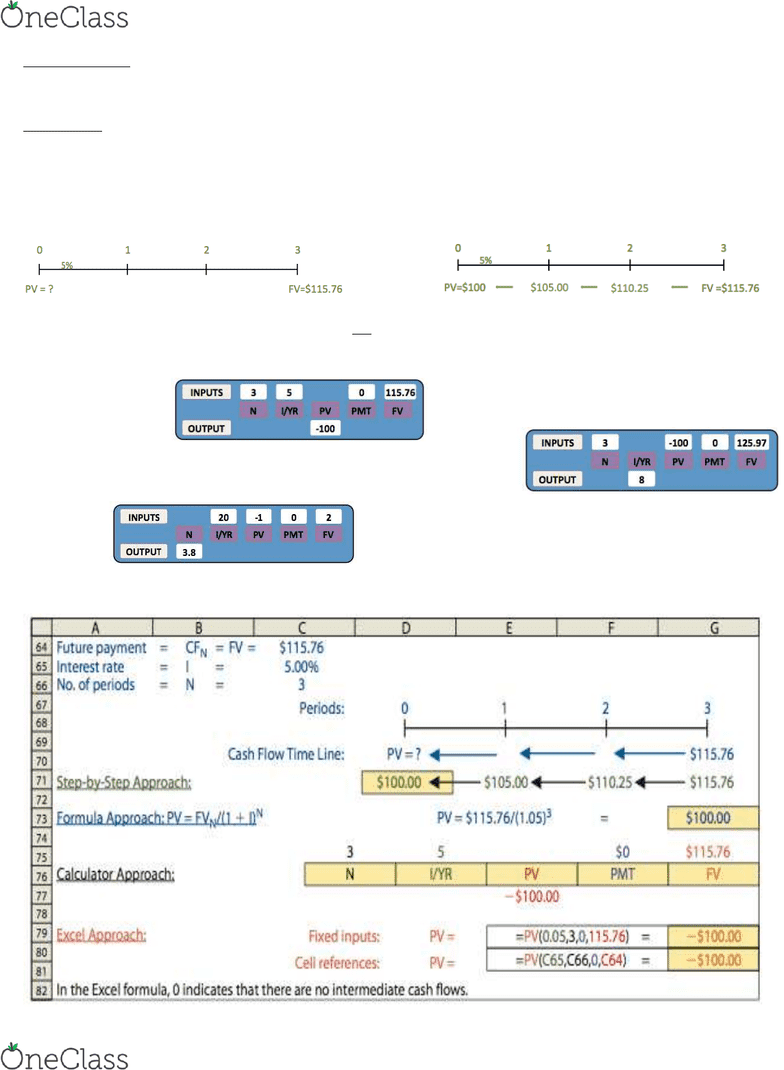Textbook Notes (280,000)
US (110,000)
NU (1,000)
FINA (60)
Chapter 5

# FINA 2201 Chapter Notes - Chapter 5: Net Present Value, Cash Flow, Interest

Department
Finance & Insurance
Course Code
FINA 2201
Professor
Ma Linlin
Chapter
5

This preview shows pages 1-2. to view the full 7 pages of the document.FINA 2201 Chapter 5: Time Value of Money
1
Timeline: An important tool used in time value analysis; it is a graphical representation used to show the timing of cash
flows.
Future Value (FV): The amount to which a cash flow or series of cash flows will grow over a given period of time when
compounded at a given interest rate.
Present Value (PV): The value today of a future cash flow or series of cash
flows.
Simple Interest: Occurs when interest is not earned on interest.
Compounding: The arithmetic processof determining the final value of a cash flow or series of cash flows when
compound interest is applied.
Compound Interest: Occurs when interest is earned on prior periods’ interest.
Future Value
You plan to deposit \$100 in a bank that pays a 5% interest each year. How
much would you have at the end of Year 3?
Finding the FV of a cash flow or series of cash flows is called compounding.
Step by step approach:
Formula approach:
Notice that we multiple (1+I) at the beginning of each period
We multiply (1+I) three times
Financial calculator
Requires 4 inputs into calculator, and will solve for the fifth.
Financial Calculators
N = Number of periods. Some calculators use n
rather than N.
I/R = Interest rate per period. Some calculators
use i or I rather than I/YR.
PV = Present value. In our example, we begin
by making a deposit, which is an outflow; so the
PV should be entered with a negative sign. On
most calculators, you must enter the 100, then
press the þ/ key to switch from þ100 to −100.
If you enter −100 directly, 100 will be subtracted
from the last number in the calculator, giving
PMT = Payment. This key is used when we
have a series of equal, or constant, payments.
Because there are no such payments in our
illustrative problem, we enter PMT 1⁄4 0. We will
use the PMT key when we discuss annuities
later in this chapter.
FV = Future value. In this example, the FV is
positive because we entered the PV as a
negative number. If we had entered the 100 as
a positive number, the FV would have been
negative.
\$1 today is worth more than a dollar in the
future because Money has time value
FVn = PV(1+i) FV3 = PV(1+i)3 = \$100(1.05)3 = \$115.76
find more resources at oneclass.com
find more resources at oneclass.com

Only pages 1-2 are available for preview. Some parts have been intentionally blurred.FINA 2201 Chapter 5: Time Value of Money
2
Opportunity Cost: The rate of return you could earn on an alternative investment of similar risk.
Present value
Discounting: The process of finding the present value of a cash flow or a series of cash flows; discounting is the reverse of
compounding.
You plan to have \$115.76 in your bank account at the end of Year 3, if the interest rate is 5%, how much you should
deposit today?
Finding the Present Value (PV) of a cash flow or series of cash flows is called discounting (the reverse of
compounding).
Step by step approach
Formula approach:
Financial Calculator
What annual interest rate would cause \$100 to grow to \$125.97 in 3 years?
If the interest rate is 20% per year, how long before one dollar to double?
PVn = PV(!+t)n Present Value = FVn
(PV) (1+ i)n
PV = FVN /(1 + I)N= FV3 /(1 + I)3
= \$115.76/(1.05)3
= \$100
find more resources at oneclass.com
find more resources at oneclass.com